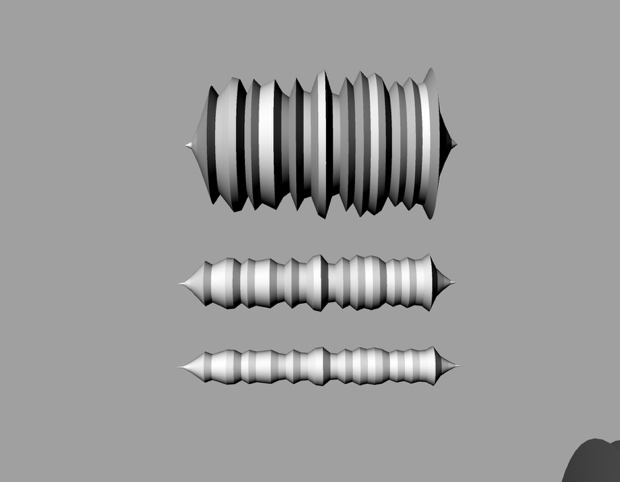# Epic Jefferson

## 10 Feb 2015How to keep your favorite sound… forever. This rhinoPython script will make your sounds printable.[sketchfab id=”8637e796380b433d994b98991077c3f7″ start=”0″ spin=”” controls=”0″]

For a long time I’ve been interested in making sounds physical in some way, I suppose in a “more tangible” way since sound is already physical. I’ve always wondered what it takes to make one of these things and I’m eager to continue to explore.

Some sketches to get the thing going.

Problems – The library I used to process the soundfile (scipy) doesn’t run from rhinoPython, so the workaround was to run a separate script for audio analysis and write the results into a file, then read that file from rhinoPython script to apply those values along the shape’s Y axis. So that particular workflow sucks, but I guess this process doesn’t have to be optimized for now.

I got my audio processing base from http://samcarcagno.altervista.org/blog/basic-sound-processing-python/

```#Analize Soundfile
from pylab import*
from scipy.io import wavfile

thickness = 160

snd = abs((snd / (2.**15)*thickness))
s1 = snd[:,0]
levels = []
points = 40

step = 5060/40
avg = 0

for i in xrange(len(s1)/step):
avg = 0
begin = i * step
end = ((i + 1) * step - 1)
temp = s1[ begin : end]

for j in xrange(len(temp)):
avg += temp[j]
avg = avg/len(temp)
levels.append(avg)

file = open("sample.txt", "w")
for i in xrange(len(levels)):
file.write(str(levels[i]))
file.write('\n')
file.close()```
```#Create Cylinder
import rhinoscriptsyntax as rs
import random

pointList = []
ypointList = []

temp = open("sample.txt", "r")
for line in temp:
ypointList.append(line)
temp.close()

for i in range(40):
Y = ypointList[i]
pt = (i, Y, 0)

pointList.append(pt)